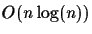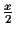Next: Introduction

# Using Traveling Salesman Problem Algorithms for Evolutionary Tree Construction

Chantal Korostensky and Gaston H. Gonnet
Institute for Scientific Computing
ETH Zurich, Switzerland
e-mail: {gonnet,korosten}@inf.ethz.ch

Date:

### Abstract:

We present a new tree construction method that constructs a tree with minimum score for a given set of sequences. To do this, the problem of tree construction is reduced to the Traveling Salesman Problem (TSP). The input for the TSP algorithm are the pairwise distances of the sequences and the output is a circular tour through the optimal, unknown tree plus the minimum score of the tree. The circular order and the score of the optimal tree can be used to construct the topology of the tree intime where n is the number of input sequences. We can guarantee that we reconstruct a correct evolutionary tree if the error for each distance measurement is smaller than, where x is the shortest edge in the tree. For data sets with large errors, a dynamic programming approach is used to reconstruct the tree.
Keywords: tree construction, Traveling Salesman, circular order, evolution

Chantal Korostensky
1999-07-14